Courses

# Physics Test 3 - Units Dimensions And Error Analysis, Kinematics, Laws Of Motion, Rotation, Gravitation, Heat And Thermodynamics

## 30 Questions MCQ Test JEE Main Mock Test Series 2020 & Previous Year Papers | Physics Test 3 - Units Dimensions And Error Analysis, Kinematics, Laws Of Motion, Rotation, Gravitation, Heat And Thermodynamics

Description
This mock test of Physics Test 3 - Units Dimensions And Error Analysis, Kinematics, Laws Of Motion, Rotation, Gravitation, Heat And Thermodynamics for JEE helps you for every JEE entrance exam. This contains 30 Multiple Choice Questions for JEE Physics Test 3 - Units Dimensions And Error Analysis, Kinematics, Laws Of Motion, Rotation, Gravitation, Heat And Thermodynamics (mcq) to study with solutions a complete question bank. The solved questions answers in this Physics Test 3 - Units Dimensions And Error Analysis, Kinematics, Laws Of Motion, Rotation, Gravitation, Heat And Thermodynamics quiz give you a good mix of easy questions and tough questions. JEE students definitely take this Physics Test 3 - Units Dimensions And Error Analysis, Kinematics, Laws Of Motion, Rotation, Gravitation, Heat And Thermodynamics exercise for a better result in the exam. You can find other Physics Test 3 - Units Dimensions And Error Analysis, Kinematics, Laws Of Motion, Rotation, Gravitation, Heat And Thermodynamics extra questions, long questions & short questions for JEE on EduRev as well by searching above.
QUESTION: 1

Solution:
QUESTION: 2

Solution:
QUESTION: 3

### The angle between vectors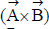and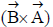is

Solution:
QUESTION: 4

A ball is thrown vertically upward with a speed v from a height h meters above the ground. The time taken for the ball to hit ground is

Solution: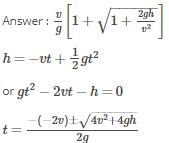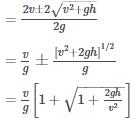Now retain only the positive sign.

QUESTION: 5
A ball rolls from the top of a stair way with a horizontal velocity u m/s. If the steps are h metre high and b metre wide, the ball will hit edge of the nth step, if
Solution: Horizontal dist. travelled=nb Vertical dist. travelled=nh nb=ut nh=0+(1/2)gt^2 => n=(2hu^2)/(gb^2)
QUESTION: 6
Which of the following is the altitude-time graph for a projectile thrown horizontally from the top of the tower?
Solution: When the time is 0 the object will be at a certain height and when we throw the object as the time increases the object will come down ie the altitude decreases.
QUESTION: 7

A long spring is stretched by 2 cm. Its potential energy is U. If the spring is streched by 10 cm, its potential energy would be

Solution:

We know, potential energy of spring is given by (1/2)kx^2 where k is spring constant and x is stretched distance.
Here , x=2cm so, U=(1/2)k*2^2=2k
For, x=10cm :-
E=(1/2)k*10^2=50k=25(2k)=25U.
Final potential energy will be 25U.

QUESTION: 8
A uniform flexible chain of mass m and length 2 l hangs in equilibrium over a smooth horizontal pin of negligible diameter. One end of the chain is given a small vertical displacement so that the chain slips over the pin.The speed of chain when it leaves pin is
Solution:
QUESTION: 9
A stone is tied with a string and is rotated in a circle horizontally. When the string suddenly breaks, the stone will move
Solution:
QUESTION: 10

A sphere A of mass m moving with constant velocity u hits another stationary sphere B of the same mass in a head on collision. if e is the coefficient of restitution, what is the ratio of velocities of sphere A to the sphere B after collision?

Solution:
QUESTION: 11
A boy of mass m is standing on a block of mass M kept on a rough surface. When the boy walks from left to right on the block, the centre of mass (boy block) of system
Solution:
QUESTION: 12

The radius of gyration of a uniform rod of length L about on axis passing through its centre of mass is

Solution:
QUESTION: 13

In case of an orbiting satellite if the radius of orbit is decreased

Solution:

KE=I w²=Iv²/r² ( since omega =v/r)
This implies that KE is inversely proportional to r²
So when radius is decreased , KE will increase due to which PE will decrease
Hence option D is correct.

QUESTION: 14
For a satellite orbiting very close to earth’s surface total energy is
Solution:
QUESTION: 15
A point P (R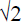,0,0) lies on the axis of a ring of mass M and radius R. The ring is located in y-z plane with its centre at origin O. A small particle of mass m starts from P and reaches O under gravitational attraction only. Its speed of O will be
Solution:
QUESTION: 16
The number of molecules per unit volume of a gas is given by
Solution:
QUESTION: 17

Pressure versus temperature graph of an ideal gas of equal number of moles of different volumes are plotted as shown in figure. Choose the correct alternative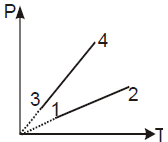Solution:
QUESTION: 18

A system undergoes a cyclic process in which it absorbs Q1 heat and gives out Q2 heat. The efficiency of the process is η and work done is W. Select the correct statement

Solution:

Concept: Carnot engine. Explanation: Heat is transferred to the working material (Q1) and heat is rejected during (Q2). The thermal efficiency is  ηth = W/Q1. Applying first law, we have,  W = Q1 − Q2.  ηth = 1 − Q2/Q1. Hence, correct answer is (b).

QUESTION: 19

A cylinder of radius R, made of a material of thermal conductivity k1,  is surrounded by a cylindrical shell of inner radius R and outer radius 2R. The shell is made of a material of thermal conductivity k2.  The two different temperatures. There is no loss of heat across the cylindrical surface and the system is in steady state. The effective thermal conductivity of the system is

Solution:
QUESTION: 20
If you set up the seventh harmonic on a string fixed at both ends,how many nodes & anti nodes are set up in it
Solution:
QUESTION: 21
A small source of sound moves on a circle as shown in figure and an observer in standing on O. Let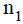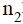and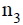be the frequencies heard when the source is at A,B and C respectively. Then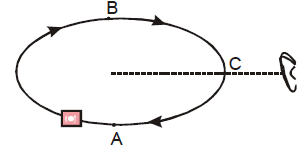Solution: At point A, source is moving away from observer so apparent frequency n1<n (actual frequency) At point B source is coming towards observer so apparent frequency n2>n and point C source is moving perpendicular to observer so n3=n Hence n2>n3>n1
QUESTION: 22
Two ends of a stretched wire of length L are fixed at x = 0 and x = L. If one experiment the displacement of the wire is y1=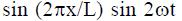and energy is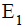and in another experiment its displacement is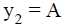and energy is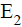, then
Solution:
QUESTION: 23
The frequency of a note emitted by a source changes by 20% as it approaches stationary observer. As it recedes away from him the apparent frequency will be different from the actual frequency by (Velocity of sound in air is 340 m/s)
Solution:
QUESTION: 24
The linear density of a vibrating string is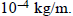A transverse wave is propagating on the string, which is described by the equation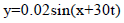where x and y are in meters and the time t is in seconds. The tension in the string is
Solution:
QUESTION: 25

Direction : Read the following question and choose

A. If both Assertion and Reason are correct and reason is the correct explanation of assertion

B. If both Assertion and Reason are true, but Reason is not correct explanation of the Assertion

C. If Assertion is true, but the Reason is false

D. If Assertion is false, but the Reason is true

E. If both assertion and reason are false

Assertion : To hear distinct beats, difference in frequencies of two sources should be less than 10
Reason : More the number of beats per sec more difficult to hear them

Solution:
QUESTION: 26

Direction : Read the following question and choose

A. If both Assertion and Reason are correct and reason is the correct explanation of assertion

B. If both Assertion and Reason are true, but Reason is not correct explanation of the Assertion

C. If Assertion is true, but the Reason is false

D. If Assertion is false, but the Reason is true

E. If both assertion and reason are false

Assertion : The bells are made of metals and not of wood
Reason : Wood offers high damping to the sound waves

Solution:
QUESTION: 27

Direction : Read the following question and choose

A. If both Assertion and Reason are correct and reason is the correct explanation of assertion

B. If both Assertion and Reason are true, but Reason is not correct explanation of the Assertion

C. If Assertion is true, but the Reason is false

D. If Assertion is false, but the Reason is true

E. If both assertion and reason are false

Assertion : For higher temperature, the peak emission wavelength of a black body shifts to lower wavelengths
Reason : Peak emission wavelength of a blackbody is proportional to the fourth power of temperature

Solution:
QUESTION: 28

Direction : Read the following question and choose

A. If both Assertion and Reason are correct and reason is the correct explanation of assertion

B. If both Assertion and Reason are true, but Reason is not correct explanation of the Assertion

C. If Assertion is true, but the Reason is false

D. If Assertion is false, but the Reason is true

E. If both assertion and reason are false

Assertion : Consider motion for a mass spring system under gravity, motion of M is not a simple harmonic motion unless Mg is negligibly small
Reason : For simple harmonic motion acceleration must be proportional to displacement and is directed towards the mean position

Solution:
QUESTION: 29

Direction : Read the following question and choose

A. If both Assertion and Reason are correct and reason is the correct explanation of assertion

B. If both Assertion and Reason are true, but Reason is not correct explanation of the Assertion

C. If Assertion is true, but the Reason is false

D. If Assertion is false, but the Reason is true

E. If both assertion and reason are false

Assertion : The principle of superposition is not valid for gravitational force
Reason : Gravitational force is a conservative force

Solution:
QUESTION: 30

Direction : Read the following question and choose

A. If both Assertion and Reason are correct and reason is the correct explanation of assertion

B. If both Assertion and Reason are true, but Reason is not correct explanation of the Assertion

C. If Assertion is true, but the Reason is false

D. If Assertion is false, but the Reason is true

E. If both assertion and reason are false

Assertion : In an elastic collision of two bodies, the momentum and energy of each body is conserved
Reason : If two bodies stick to each other, after collision, the collision is said to be perfectly elastic

Solution: